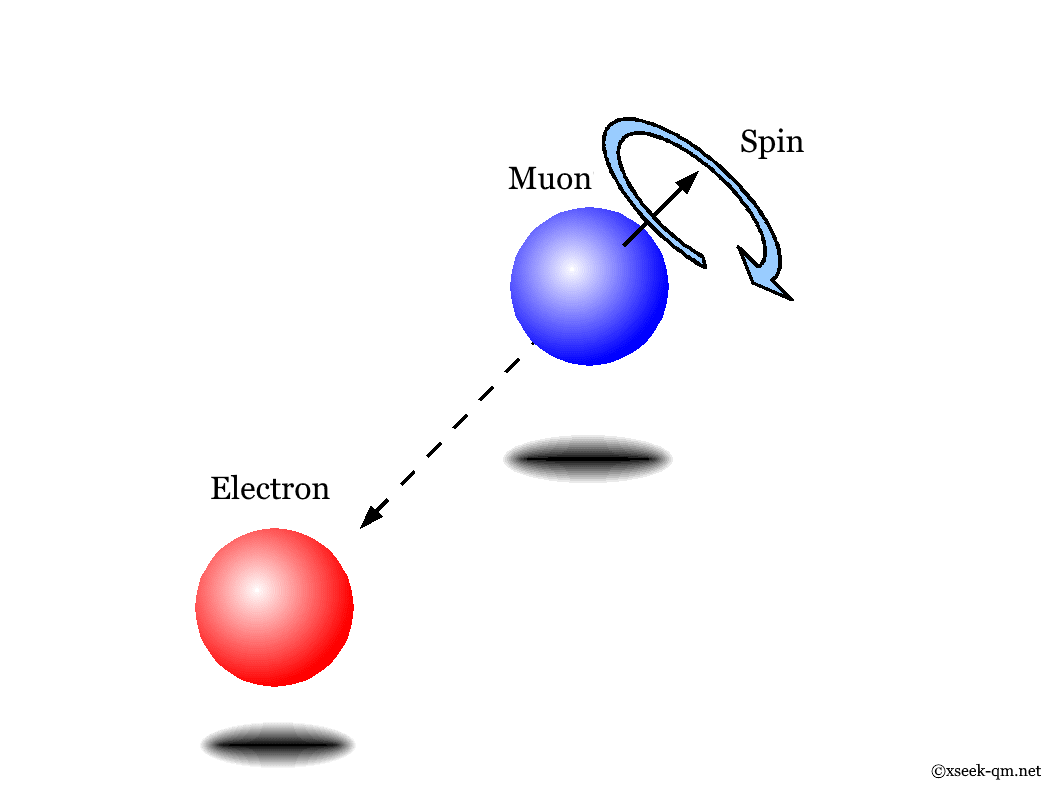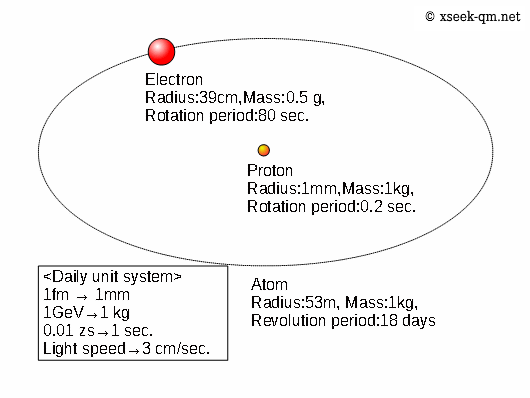# Spin of quantum mechanics

2014/9/1
Published 2002/3/9

The spin of the quantum mechanics is a spin of a small particle like an electron.## What is spin?

The water, which we daily drink is made of hydrogen atoms and oxygen atoms. With respect to hydrogen atoms, an electron revolves around a proton. It is very difficult for us to imagine the atom because it is very small. Therefore, I would like to express the atom in the unit system to use daily. We call the unit system "daily unit system."In the above figure, though the radius of the electron is 390 femtometers (daily unit:39 centimeters). However, it is not correct in fact, because it is experimentally confirmed that the electron is smaller than 1 femtometer (daily unit: 1 millimeter).

The proton and the electron are spinning. In quantum mechanics, we call it spin. The particle neither speed up, nor slow down the rotational velocity of the spin. The angular momentum of the spin is fixed.

In order to explain the spin, we consider the spin of a mu particle. The mu particle is a particle like an electron. The mass is 106 MeV (daily unit: 106g), and the lifetime is two microsecond (daily unit: 700 million years). The mu particle decays into two neutrinos and an electron.The axis of rotation of the spin has any direction without magnetic field. We will find the direction of the axis of rotation of the spin by observing the electron popping from the mu particle because the electron pops in the opposite direction of the spin of the mu particle.

On the other hand, the spin has only two directions with magnetic field. The state of the spin is a superposition of the state that the direction of the spin is the same direction of the magnetic field and the direction of the spin is the opposite direction of the magnetic field. The direction of the popping electron from the mu particle is only the two directions. In other words, the magnetic field breaks the rotational symmetry.

## Is the spin rotation?

A lot of people do not think the spin is rotation because there are the following two problems.

### Rotational velocity

It is experimentally confirmed that the radius of the electron is smaller than 1 femtometer (daily unit: 1 millimeter). To realize the momentum of the spin the rotational velocity of the spin becomes 390 times of the light speed. If the radius of the electron is 390 femtometers (daily unit: 39 centimeters) the rotational velocity of the spin becomes the light speed.

### Two-valuedness of the wave function

If we rotate the spin 360 degrees, the phase of the wave function will be reversed. If we rotated the spin 720 degrees, to return to the original phase the wave function. Therefore, the wave function of the spin has two-valuedness.

## Summary

Due to the above problems, a lot of people do not think the spin is rotation. In order to interpret the spin as the rotation I wrote the following paper.

I hope this paper helps you to interpret the spin as the rotation.

Related Articles: